Function Repository Resource:

# NetFuseBatchNorms

Fuse a BatchNormalization layer preceded by a ConvolutionLayer into a single ConvolutionLayer

Contributed by: Maria Sargsyan
 ResourceFunction["NetFuseBatchNorms"][convLayer, bnLayer] fuses the weights of an initialized BatchNormalizationLayer and ConvolutionLayer into a single ConvolutionLayer whenever possible. ResourceFunction["NetFuseBatchNorms"][net] repeatedly fuses the weights of an initialized BatchNormalizationLayer preceded by an initialized ConvolutionLayer into a single ConvolutionLayer in a net.

## Details

ResourceFunction["NetFuseBatchNorms"] does not do anything on an uninitialized net.

## Examples

### Basic Examples (6)

Define a non-zero initialized ConvolutionLayer followed by a BatchNormalizationLayer:

 In:=Out=In:=Out=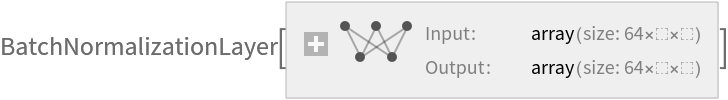Fuse the convLayer and bnLayer into a single ConvolutionLayer:

 In:=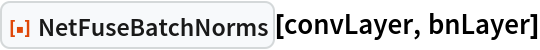Out=Create a NetChain:

 In:=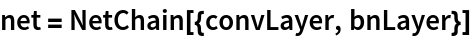Out=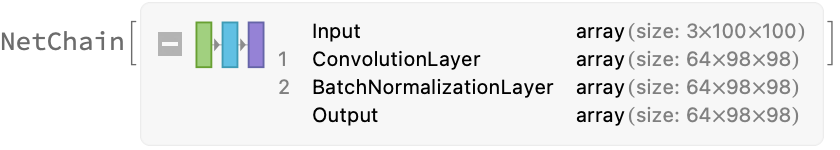Perform the same combination within a NetChain:

 In:=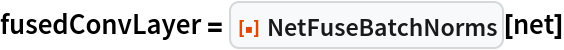Out=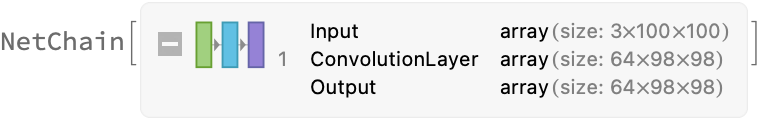Compare the outputs on a random input:

 In:=Out=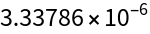Note that the fused layer is faster:

 In:=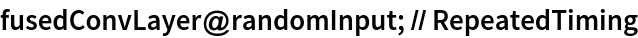Out=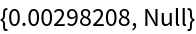In:=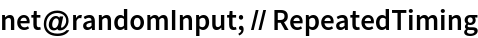Out=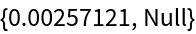### Scope (1)

Note that NetFuseBatchNorms does not do anything on an uninitialized net:

 In:=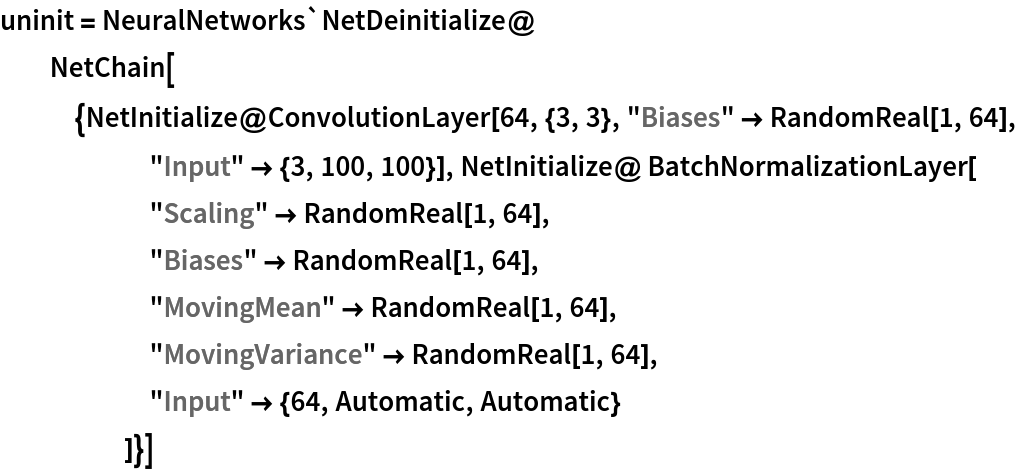Out=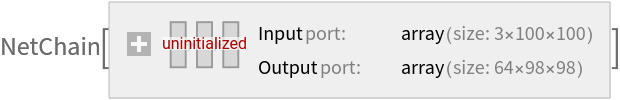In:=Out=In:=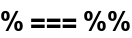Out=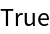### Applications (4)

Get pretrained ShuffleNet-V2:

 In:=Accelerate ShuffleNet-V2:

 In:=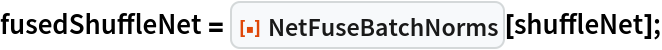Compare the outputs on a random image:

 In:=Out=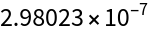The fused net is lighter as it contains less BatchNormalizationLayer layers:

 In:=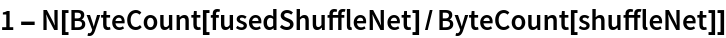Out=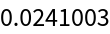In:=Out=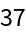## Version History

• 1.0.0 – 08 December 2022